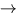# Choosing a Coordinate System

Idea: When solving problems with potential energy, choosing the origin of the coordinate system is equivalent to choosing the place where the potential energy is zero. We know that the physics must be independent of the choice of coordinate systemthe value of the potential energy at any given place has no physical significance. The quantity that does have physical significance is the change in PE from one position to another.

Usual Choices:

1.
For PEg we usually choose the origin of the coordinate system at the point where the motion originates. For Example: If a stone is thrown upward from the surface of the earth, we choose y = 0 at the surface of the earth which means PEg = 0 at the surface of the earth. If the stone is thrown from the top of a building, we choose y = 0 at the top of the building. Then the stone has some negative value of PEg when it reaches the ground.
2.
For PEs we usually choose x = 0 at the equilibrium position of the spring (where the spring is neither stretched or compressed).Next: Conservation Laws Up: Work and Energy Previous: Potential Energy Stored in